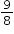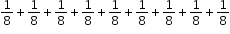# MA.3.FR.1.2

Represent and interpret fractions, including fractions greater than one, in the form of $\frac{m}{n}$ as the result of adding the unit fraction $\frac{1}{n}$ to itself times.

### Examplescan be represented as.

### Clarifications

Clarification 1: Instruction emphasizes conceptual understanding through the use of manipulatives or visual models, including circle graphs, to represent fractions.

Clarification 2: Denominators are limited to 2, 3, 4, 5, 6, 8, 10 and 12.

General Information
Subject Area: Mathematics (B.E.S.T.)
Strand: Fractions
Date Adopted or Revised: 08/20
Status: State Board Approved

## Related Courses

This benchmark is part of these courses.
5012050: Grade Three Mathematics (Specifically in versions: 2014 - 2015, 2015 - 2022, 2022 and beyond (current))
7712040: Access Mathematics Grade 3 (Specifically in versions: 2014 - 2015, 2015 - 2018, 2018 - 2022, 2022 and beyond (current))
5012055: Grade 3 Accelerated Mathematics (Specifically in versions: 2019 - 2022, 2022 and beyond (current))
5012015: Foundational Skills in Mathematics 3-5 (Specifically in versions: 2019 - 2022, 2022 and beyond (current))

## Related Access Points

Alternate version of this benchmark for students with significant cognitive disabilities.
MA.3.FR.1.AP.2: Explore fractions, less than or equal to a whole, in the form of m/n as the result of adding the unit fraction 1/n to itself m times. Denominators are limited to 2, 3 and 4.

## Related Resources

Vetted resources educators can use to teach the concepts and skills in this benchmark.

## Formative Assessments

Three Quarters Of The Race:

Students are read a word problem about a student who has run three-fourths of a race and asked to describe what that means.

Type: Formative Assessment

Painting A Wall:

Students are read a word problem about a wall being painted and asked to describe what three-eighths of the wall means.

Type: Formative Assessment

How Many Fourths Are In Two Wholes?:

Students are asked to divide two rectangles into fourths and then to consider how many fourths the two wholes represent.

Type: Formative Assessment

## Image/Photograph

Clipart ETC Fractions:

Illustrations that can be used for teaching and demonstrating fractions. Fractional representations are modeled in wedges of circles ("pieces of pie") and parts of polygons. There are also clipart images of numerical fractions, both proper and improper, from halves to twelfths. Fraction charts and fraction strips found in this collection can be used as manipulatives and are ready to print for classroom use.

Type: Image/Photograph

## Lesson Plan

Fraction Action!:

This lesson will help students understand that fractions are parts of a whole. The lesson introduces fractional parts using familiar manipulatives.

Type: Lesson Plan

## Original Student Tutorials

Fraction Camp! Fractions Greater Than 1 on a Number Line:

Joey uses his knowledge of fractions to win games at camp by knowing where fractions greater than one are located on number lines, in this interactive tutorial.

Type: Original Student Tutorial

Nature Walk: Fractions Less Than 1 on a Number Line:

Learn to use number lines to represent fractions as Emmy explores nature in this interactive tutorial.

Type: Original Student Tutorial

## Virtual Manipulative

Build a Fraction:

This virtual manipulative will help the students to build fractions from shapes and numbers to earn stars in this fraction lab. To challenge the children there are multiple levels, where they can earn lots of stars.
Some of the sample learning goals can be:

• Build equivalent fractions using numbers and pictures.
• Compare fractions using numbers and patterns
• Recognize equivalent simplified and unsimplified fractions

Type: Virtual Manipulative

## MFAS Formative Assessments

How Many Fourths Are In Two Wholes?:

Students are asked to divide two rectangles into fourths and then to consider how many fourths the two wholes represent.

Painting A Wall:

Students are read a word problem about a wall being painted and asked to describe what three-eighths of the wall means.

Three Quarters Of The Race:

Students are read a word problem about a student who has run three-fourths of a race and asked to describe what that means.

## Original Student Tutorials Mathematics - Grades K-5

Fraction Camp! Fractions Greater Than 1 on a Number Line:

Joey uses his knowledge of fractions to win games at camp by knowing where fractions greater than one are located on number lines, in this interactive tutorial.

Nature Walk: Fractions Less Than 1 on a Number Line:

Learn to use number lines to represent fractions as Emmy explores nature in this interactive tutorial.

## Student Resources

Vetted resources students can use to learn the concepts and skills in this benchmark.

## Original Student Tutorials

Fraction Camp! Fractions Greater Than 1 on a Number Line:

Joey uses his knowledge of fractions to win games at camp by knowing where fractions greater than one are located on number lines, in this interactive tutorial.

Type: Original Student Tutorial

Nature Walk: Fractions Less Than 1 on a Number Line:

Learn to use number lines to represent fractions as Emmy explores nature in this interactive tutorial.

Type: Original Student Tutorial

## Virtual Manipulative

Build a Fraction:

This virtual manipulative will help the students to build fractions from shapes and numbers to earn stars in this fraction lab. To challenge the children there are multiple levels, where they can earn lots of stars.
Some of the sample learning goals can be:

• Build equivalent fractions using numbers and pictures.
• Compare fractions using numbers and patterns
• Recognize equivalent simplified and unsimplified fractions

Type: Virtual Manipulative

## Parent Resources

Vetted resources caregivers can use to help students learn the concepts and skills in this benchmark.

## Image/Photograph

Clipart ETC Fractions:

Illustrations that can be used for teaching and demonstrating fractions. Fractional representations are modeled in wedges of circles ("pieces of pie") and parts of polygons. There are also clipart images of numerical fractions, both proper and improper, from halves to twelfths. Fraction charts and fraction strips found in this collection can be used as manipulatives and are ready to print for classroom use.

Type: Image/Photograph

## Virtual Manipulative

Build a Fraction:

This virtual manipulative will help the students to build fractions from shapes and numbers to earn stars in this fraction lab. To challenge the children there are multiple levels, where they can earn lots of stars.
Some of the sample learning goals can be:

• Build equivalent fractions using numbers and pictures.
• Compare fractions using numbers and patterns
• Recognize equivalent simplified and unsimplified fractions

Type: Virtual Manipulative